#### 期刊菜单

Analytical Method of Luffing Character of Lemniscate Type Crane with Boom-Driving
DOI: 10.12677/MET.2022.116076, PDF, HTML, XML, 下载: 33  浏览: 77

Abstract: The luffing mechanism of a foreign company’s crane is designed elaborately and patent is accredit-ed. There are great differences in outward appearance, geometric shape, luffing performance of lemniscate type crane. Based on theory of mechanism and inverse engineering, luffing mechanism characters of lemniscate type crane are studied, luffing moment is analyzed. By sample analysis, the luffing mechanism of lemniscate type crane has good kinematic characteristic and luffing operation.

1. 引言

2. 双纽线型变幅机构运动学分析Figure 1. Photo of lemniscate luffing mechanism (with boom driving)

2.1. 速度瞬心P求解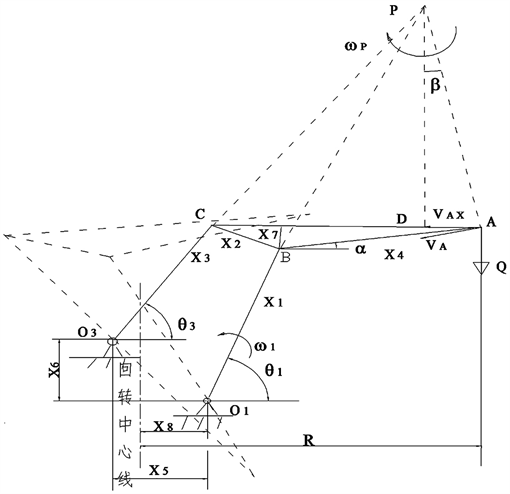X1——臂架(主动件)；X2——象鼻架后臂；X3——后摇杆；X4——象鼻架前臂；X5——后摇杆下铰点O3与臂架下铰点O1之水平距离；X6——后摇杆下铰点O3与臂架下铰点O1之铅垂距离；X7——象鼻架的下沉量；X8——回转中心线与臂架下铰点O1之水平距离；R——幅度；γ——臂架与象鼻架后臂之夹角；θ1——臂架与水平线之夹角(自变量)；θ3——后摇杆与水平线之夹角；β——A点速度向量与水平线之夹角；β0——象鼻架前臂与后臂之夹角；α——象鼻架前臂与水平线之夹角；ω1——驱动臂架之角速度；Q——起重量。

Figure 2. Schematic diagram of luffing mechanism of lemniscate type crane with boom driving

1) ${\theta }_{1}<{90}^{\circ }$

$\left(\text{I}\right)\text{\hspace{0.17em}}\text{\hspace{0.17em}}{\theta }_{1}-{\theta }_{3}>0,\text{\hspace{0.17em}}\text{\hspace{0.17em}}\stackrel{¯}{{O}_{1}P}={X}_{56}\mathrm{sin}\left({\theta }_{3}+{\theta }_{0}\right)/\mathrm{sin}\left({\theta }_{1}-{\theta }_{3}\right)$ (1)

${X}_{56}=\sqrt{{X}_{5}^{2}+{X}_{6}^{2}};\text{\hspace{0.17em}}\text{\hspace{0.17em}}{\theta }_{0}=\mathrm{arctan}\left({X}_{6}/{X}_{5}\right)$.

2) ${\theta }_{1}>{90}^{\circ }$

$\left(\text{II}\right)\text{\hspace{0.17em}}\text{\hspace{0.17em}}{\theta }_{1}-{\theta }_{3}<0,\text{\hspace{0.17em}}\text{\hspace{0.17em}}\stackrel{¯}{{O}_{1}P}={X}_{56}\mathrm{sin}\left({\theta }_{3}+{\theta }_{0}\right)/\mathrm{sin}\left({\theta }_{3}-{\theta }_{1}\right)$ (2)

${X}_{56}=\sqrt{{X}_{5}^{2}+{X}_{6}^{2}};\text{}{\theta }_{0}=\mathrm{arctan}\left({X}_{6}/{X}_{5}\right)$.

2.2. ${\theta }_{3}=f\left({\theta }_{1}\right)$ 函数关系的求解

$\stackrel{\to }{{O}_{1}{O}_{3}}+\stackrel{\to }{{O}_{3}C}=\stackrel{\to }{{O}_{1}B}+\stackrel{\to }{BC}\text{\hspace{0.17em}}$ (3)

$\left\{\begin{array}{l}{X}_{3}^{2}+{X}_{56}^{2}-2{X}_{56}{X}_{3}\mathrm{cos}\left({\theta }_{3}+{\theta }_{0}\right)={Y}^{2}\\ {X}_{56}^{2}+{X}_{1}^{2}-2{X}_{56}{X}_{1}\mathrm{cos}\left(\text{π}-{\theta }_{1}-{\theta }_{0}\right)={L}^{2}\end{array}$ (4)

YL——中间量。

${\theta }_{3}=a\mathrm{cos}\frac{{X}_{3}^{2}+{L}^{2}-{X}_{2}^{2}}{2{X}_{3}L}+a\mathrm{cos}\frac{{X}_{56}^{2}+{L}^{2}-{X}_{1}^{2}}{2{X}_{56}L}-{\theta }_{0}$ (5)

2.3. 象鼻架前端仰角α的求解

$\alpha =\text{π}-{\beta }_{0}-a\mathrm{cos}\frac{{X}_{1}^{2}+{X}_{2}^{2}-{Y}^{2}}{2{X}_{1}{X}_{2}}+{\theta }_{1}$ (6)

${\beta }_{0}=a\mathrm{cos}\frac{{X}_{2}^{2}+{X}_{4}^{2}-C{A}^{2}}{{X}_{2}{X}_{4}}$.

2.4. 象鼻架轴心线与水平线夹角α1的求解

${\alpha }_{1}={\theta }_{1}+a\mathrm{sin}\frac{{X}_{7}}{{X}_{2}}-a\mathrm{cos}\frac{{X}_{1}^{2}+{X}_{2}^{2}-{Y}^{2}}{2{X}_{1}{X}_{2}}$ (7)

Y——见式(4)。

3. 水平分速度的求解

3.1. 吊点A的运动轨迹方程

$\left\{\begin{array}{l}{X}_{A}={X}_{1}\mathrm{cos}{\theta }_{1}+{X}_{4}\mathrm{cos}\alpha +{X}_{8}\\ {Y}_{A}={X}_{1}\mathrm{sin}{\theta }_{1}+{X}_{4}\mathrm{sin}\alpha \end{array}$ (8)

α——见式(6)。

3.2. 吊点A的水平分速度求解

$\begin{array}{l}{V}_{\text{B}}={\omega }_{1}\cdot \stackrel{¯}{{O}_{1}B}={\omega }_{P}\cdot \stackrel{¯}{PB}\\ {\omega }_{P}={\omega }_{1}\cdot \stackrel{¯}{{O}_{1}B}/\stackrel{¯}{PB}\end{array}$ (9)

$\begin{array}{l}{X}_{1}=\stackrel{¯}{{O}_{1}B},\text{}\mathrm{cos}\beta =\stackrel{¯}{PD}/\stackrel{¯}{PA}\\ {V}_{A}={\omega }_{P}\cdot \stackrel{¯}{PA}\\ {V}_{AX}={V}_{A}\cdot \mathrm{cos}\beta ={\omega }_{1}\cdot {X}_{1}\cdot \stackrel{¯}{PD}/\stackrel{¯}{PB}\end{array}$ (10)

4. 变幅力矩的求解

${M}_{Q}=Q\cdot \stackrel{¯}{DA}\cdot \stackrel{¯}{{O}_{1}B}/\stackrel{¯}{PB}=Q\cdot {X}_{1}\cdot \stackrel{¯}{DA}/\stackrel{¯}{PB}$ (11)

5. 分析计算实例Table 1. Results of analysis and calculation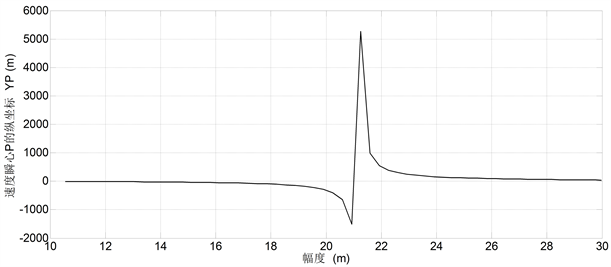Figure 3. Locus of velocity instantaneous center P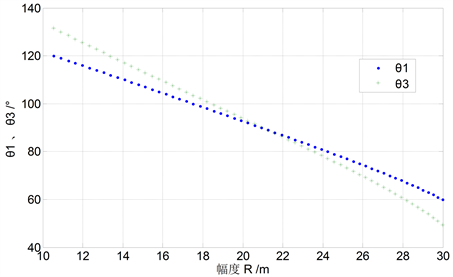Figure 4. Relationship curve of ${\theta }_{3}=f\left( \theta 1 \right)$Figure 5. Curve of α1 between flying jib axis and horizontal line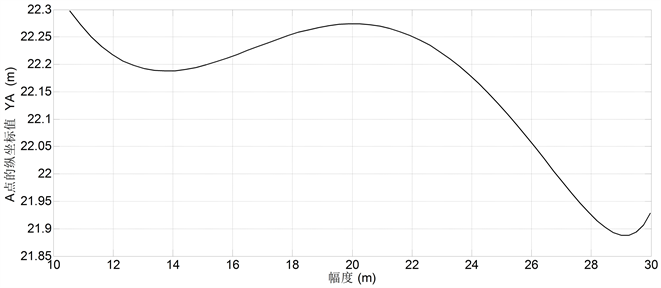Figure 6. Curve of luffing movement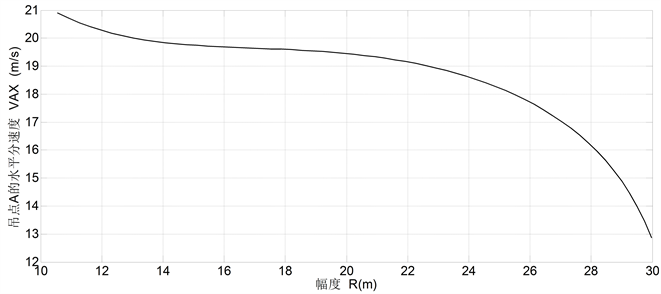Figure 7. Curve of horizontal velocity of point A (supposed ω1 = 1)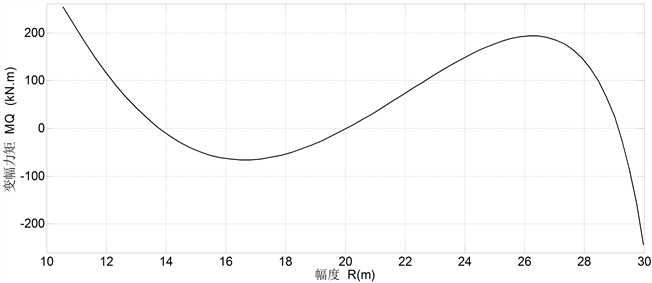Figure 8. Diagram of luffing moment MQ

6. 结论

1) 该型变幅机构的变幅运动轨迹近似为水平线，落差仅仅0.4098 m；其相对水平度为

$\frac{0.4098}{30-10.5}×100%=2.10%$

2) 与后摇杆驱动双纽线型起重机  比较，其变幅过程的水平分速度约增大30%，对装卸转载作业有利；

3) 与后摇杆驱动双纽线型起重机  比较，其变幅力矩约增大10%~30%；

4) 该变幅机构可以推广应用于机器人的工作机构。

  Kenz Figee B.V. Company (2011) Brochure of Kenz-Figee: Offshore & Harbour Cranes. http://www.Kenz-Figee.com  Nieuwenhuis, G. and Dorresteijn, J.J. (2000) Crane. European Patent No. EP1048606A1.  李克勤, 姜翠香. 一种新型四连杆变幅机构的反求与MATLAB实现[J]. 湖北工业大学学报, 2008, 23(3): 76-77, 80.  李克勤, 姜翠香. 后摇杆驱动臂架起重机的变幅机构反求设计[J]. 起重运输机械, 2002(5): 8-9.  李克勤, 姜翠香. 臂架驱动双纽线型起重机变幅机构的受力分析和实现[J]. 中国工程机械学报, 2017, 15(5): 447-451.  李克勤, 姜翠香. 双纽线型起重机两种类型变幅机构的比较[J]. 中国工程机械学报, 2016, 14(1): 50-53.  李克勤. 后摇杆驱动臂架型起重机变幅系统分析与优化设计的研究[D]: [硕士学位论文]. 武汉: 武汉理工大学, 1993.  李克勤. 具有近似水平直线运动轨迹双摇杆变幅机构的运动学分析与计算[J]. 港口装卸, 2002(2): 5-7.  Li, K.Q. and Jiang, C.X. (2009) Inverse Design of a New Double-Link Luffing Mechanism and Realization on MATLAB. In: Proceedings of the 3rd International Conference on Mechanical Engineering and Mechanics, Science Press USA Inc., Monmouth Junction, 301-304.  李克勤, 姜翠香. 新型后摇杆驱动四连杆变幅机构的传力特性与变幅力矩分析[J]. 科学技术与工程, 2011, 11(35): 8723-8725, 8730.  李克勤, 姜翠香. 新型后摇杆驱动四连杆变幅机构的受力分析与程序实现[J]. 科学技术与工程, 2012, 12(13): 3231-3234.  李克勤. 后摇杆驱动臂架型起重机的变幅机构运动学与受力分析软件V1.0 [P]. 中国专利, 2014SR153105. 2014.  Li, K.Q. and Li, J.N. (2012) Kinematics and Luffing Moment of Lemniscate Type Crane with Boom Driving. Advanced Materials Research, 503-504, 923-926. https://doi.org/10.4028/www.scientific.net/AMR.503-504.923  Li, K.Q. and Li, J.N. (2012) Force Analysis of Luff-ing Mechanism of Lemniscate Type Crane with Boom Driving. Advanced Materials Research, 538-541, 2618-2621.  李克勤. 双纽线型起重机的变幅机构运动分析软件V1.0 [P]. 中国专利, 2015SR026039. 2015.  Uicker, J.J., Pennock, G.R. and Shigley, J.E. (2010) Theory of Machines and Mechanisms. 4th Edition, Oxford University Press, Oxford.